#Function Repository Resource:

# SimpleHypergraphPlot

Plot a hypergraph defined by a list of hyperedges and isolated vertices

Contributed by: Daniel McDonald
 ResourceFunction["SimpleHypergraphPlot"][list] plots the hypergraph defined by list.

## Details and Options

The hypergraph represented by {{v11,v12,},{v21,v22,},,u1,u2,} has vertices v11,v12,,v21,v22,,,u1,u2, and hyperedges {v11,v12,},{v21,v22,},.

## Examples

### Basic Examples

Plot the hypergraph having vertices a, b, c, d, e, f, g and hyperedges {a,b,c,d}, {b,c}, {c,d,e}:

 In:=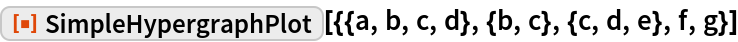Out=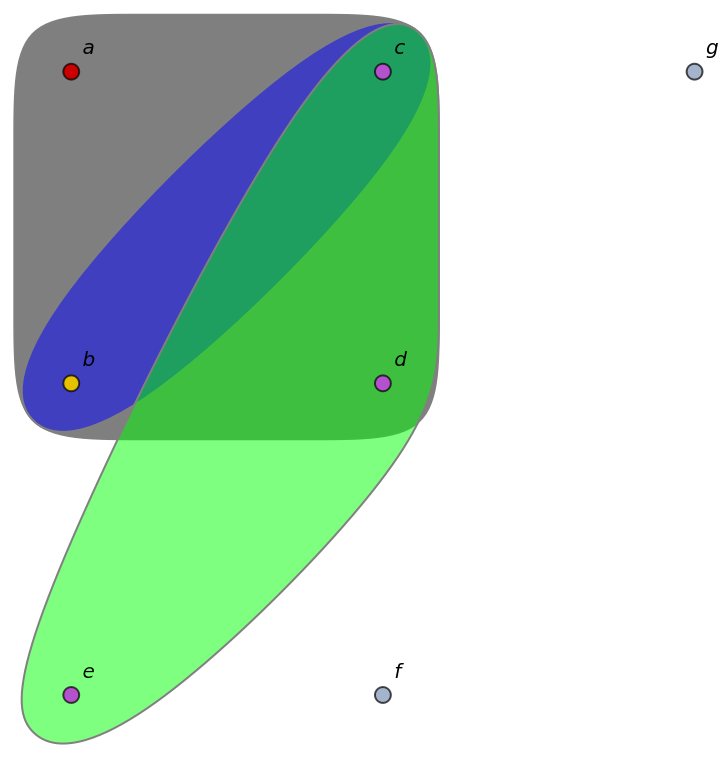### Applications

Define a random hypergraph having n vertices and m hyperedges:

 In:=Out=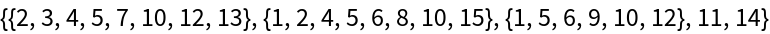Plot it:

 In:=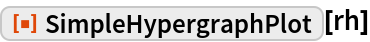Out=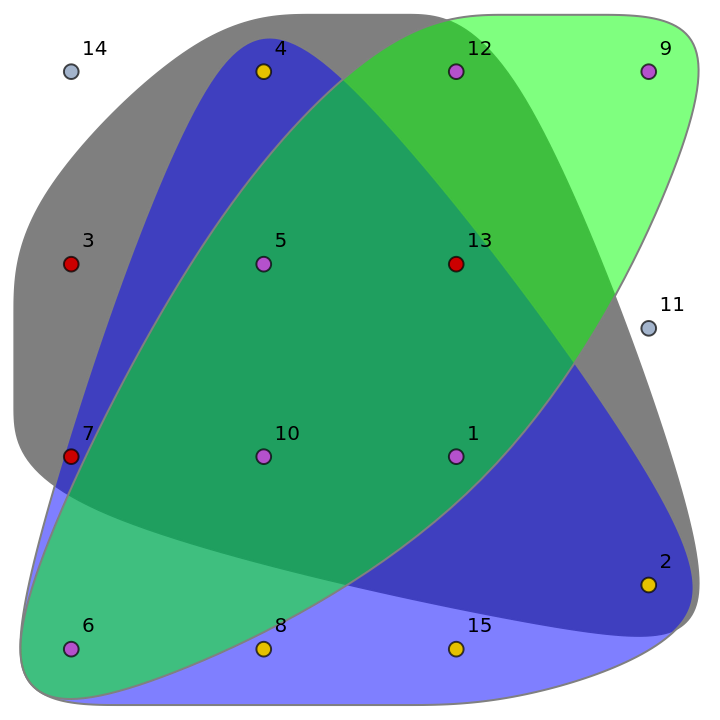## Author Notes

This function was originally published under the name HypergraphPlot